# Xilinx FFT core generator - IFFT with symmetric input

Status
Not open for further replies.

#### Knyquist

##### Newbie level 5
Hello!
I'm dealing with the FFT core from Xilinx.
What I would like to do is to take a real signal in input, perform its fft and then reconvert it into a real signal by doing its ifft.

I'm facing a problem regarding the ifft. Actually, even if the output signal from the first fft block is symmetrical (complex conjugate) what I obtain
at the outtput of its ifft is something weird.

The set up is the following:
Radix 2 Burst - Fixed point 19 bit transform with 256 points and natural order output.

Here there are a few graphs to explain what happens. I'm plotting them with matlab because I've set up my vhdl so that to write files with output and input data during the several stages of the transform

The input signal is formed by the sum of two sinusoids, the first one with two entire periods within 256 samples, the second one with 4 periods. So the input is shown here: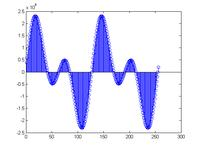the fft block perform its transformation correctly and at the output i obtain these grapsh for the real part and imaginary part respectively: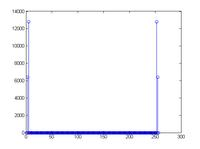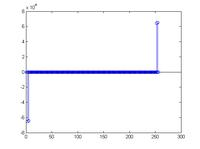These graphs are correct because peaks are presented at k= 2,4,252,254

Now, if I take the ifft in matplab of these data what I obtain is exactly the input signal (its real part, the imaginary part is null) (with a scale factor)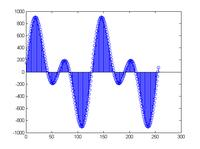While at the output of the ifft implementation in vhdl I obtain these results for the real part and imaginary part: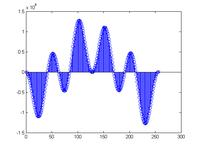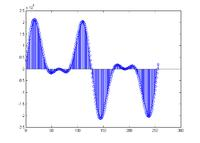Of course what I would like to obtain is a pure real signal.

A considerations:
- I'm aware about Jim Wu's Blog post about how to get real output from ifft: https://myfpgablog.blogspot.it/2011/04/ifft-with-symmetric-input-in-system.html
My X(0) and X(128) samples from fft are real (I impose them to be real), thus this doesn't work for me. (Or maybe I didn't get the point).

Any help will be welcomed!
Thanks and best regards!

What about the spectrum of the signal you get from the "wrong" ifft? Do it with matlab..

Actually that was a good point.
Here it is (real part and imaginary part):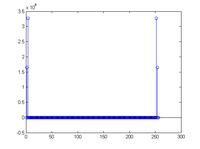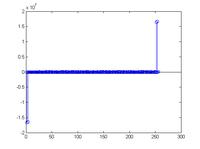They are very similar except the fact that, if we take a close look to the end of the transform (real part):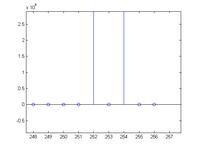as you can see it seems as if the input data was not scheduled properly inside the ifft module (shifted right by 1 bin).
I was implicitly assuming a correct data scheduling at the input because I checked the waveforms a lot of times, and here they are actually: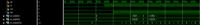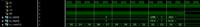the samples are provided correctly, according to the indexes and the rfd signals.
It seems like even if the timings are respected it doesn't samples the first one.
In any case, I guess it's due to a bad schedule of my command signals.

Anyway. I'll come back soon with further data.

Last edited:

Status
Not open for further replies.